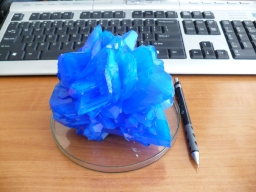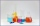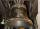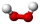# Copper sulphate

How much g of water do we have to add to 240 g of an 84% CuSO4 solution to produce a 60% solution? (Express the mass of crystalline CuSO4 in the original solution and in the resulting solution and compare them. )

w =  96 g
m1 =  201.6 g
m2 =  201.6 g

### Step-by-step explanation:

0.84•240 = 0.60 •(240+w)

0.6w = 57.6

w = 96

Our simple equation calculator calculates it.Did you find an error or inaccuracy? Feel free to write us. Thank you!Tips to related online calculators
Do you have a linear equation or system of equations and looking for its solution? Or do you have a quadratic equation?

## Related math problems and questions:

• SolutionsHow much 60% solution and how much 35% solution is needed to create 100 l of 40% solution?
• Alcohol solutionsWe have to produce 2 liters of 60% alcohol from 55% and 80%. How many of which ones will we use in the solution?
• ConcentrationHow many grams of 65% and 50% acid is to be mixed to give 240 g acid with a concentration of 60%?
• SolutionWhat amount of 80% solution and what amount of water is needed to mix in order to obtain two liters of 60% solution of this mixture?
• CuSO4 mixtureHow many grams of solid CuSO4 we have to add to 450g of 15% CuSO4 solution to produce a 25% solution?
• GramsHow many grams of 5% solution do we need to add to 100g of 50% solution to get a 20% solution?
• Alcohol mixtureFrom 55% and 80% alcohol, we have to produce 0.2 kg of 60% alcohol. How many of them do we use in the solution?
• SpiritFrom 55% and 80% spirit we would like to produce 0.2 kg of 60% spirit. How many of them we must use in a solution?
• Solutions, mixturesHow many liters of 70% solution we must add to 5 liters of 30% solution to give us a 60% solution?
• Water solutionHow many grams of salt should we dissolve in 400 g of water to get a 20% solution?
• Alcohol mixingHow much 64% of the alcohol must be poured into 6 liters of 85% alcohol to produce 79% alcohol?
• Bronze, tin and copperBronze is an alloy of tin and copper. An alloy of 10% tin and 90% copper is Gunmetal. If it contains 20% tin and 80% copper, it is bell metal. How many tons of molten bell metal and how many tons of copper is needed to make 100 tons of Gunmetal?
• Solutions, mixturesWe have 2 liters of 20% solution available. How much 70% solution do we need to add to it to get a 30% solution?
• Acid solutionBy adding 250 grams of a 96% sulfuric acid solution to its 3% solution, we changed its initial concentration to 25%. How many grams of 3% of the acid was used for dilution?
• Acid evaporationHow many kilograms of water do we have to evaporate from 100 kg of 32% acid to make it 80% concentration?
• CloggingHow much-distilled water must the pharmacist add to 30g of a 30% hydrogen peroxide solution to obtain a 3% solution to clogging?
• Disinfecting solutionHow much distilled water is necessary to pour into 500 ml of 33% hydrogen peroxide solution to obtain 3% disinfecting solution?